Addition & Subtraction Within 1,000 Reading Material | Generation Genius
1%
It was processed successfully!

# Addition & Subtraction Within 1,000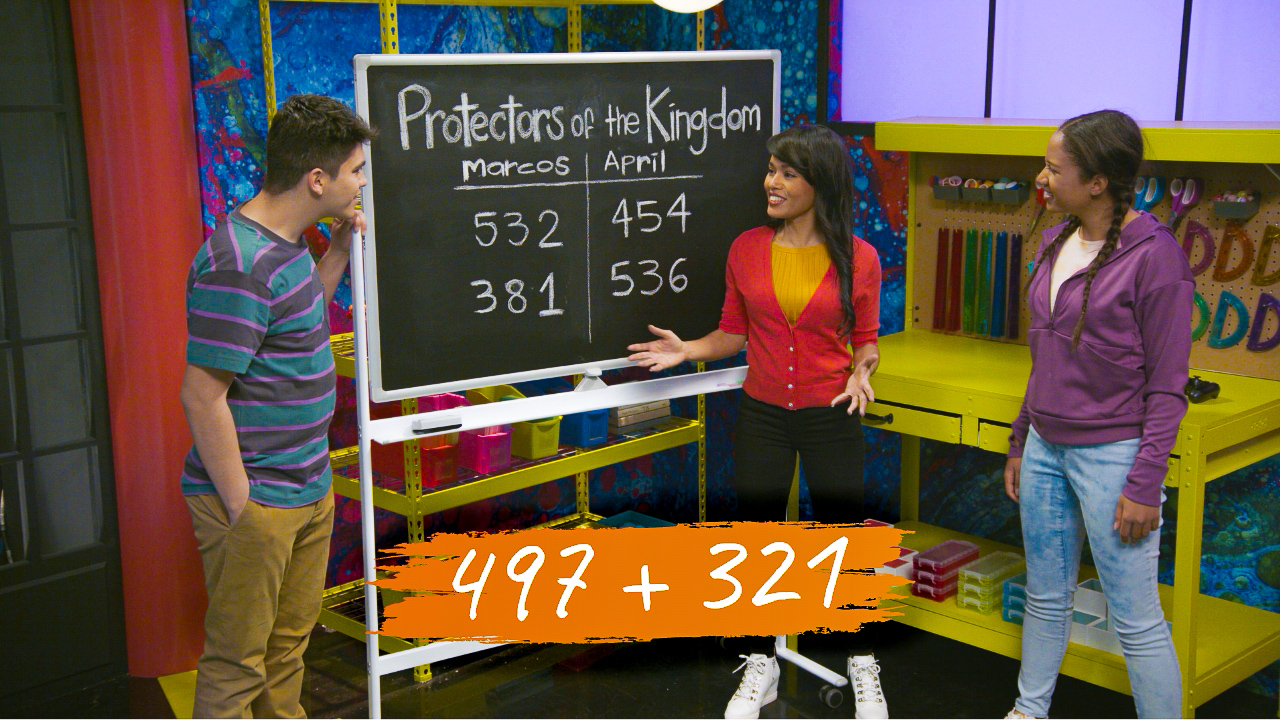WHAT IS ADDITION & SUBTRACTION WITHIN 1,000?

Students learn and understand the process of adding and subtracting 3-digit numbers by using models such as base-10 blocks and number lines. Place value is emphasized in understanding adding or subtracting within each part of the numbers, and in understanding the relationship between each place when regrouping.

To better understand addition & subtraction within 1,000…

WHAT IS ADDITION & SUBTRACTION WITHIN 1,000?. Students learn and understand the process of adding and subtracting 3-digit numbers by using models such as base-10 blocks and number lines. Place value is emphasized in understanding adding or subtracting within each part of the numbers, and in understanding the relationship between each place when regrouping. To better understand addition & subtraction within 1,000…

## LET’S BREAK IT DOWN!

### Weightlifting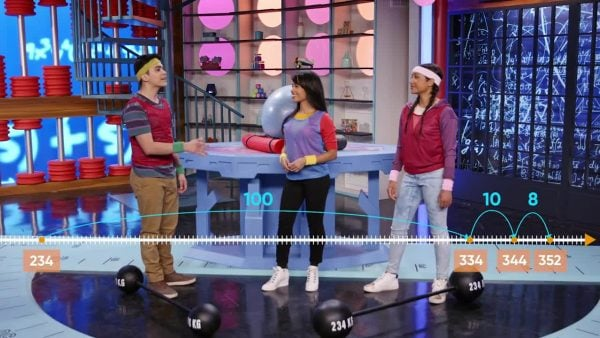Adding 3-digit numbers can help you find the total weight two people can lift. Let’s say Marco can lift 118 kg and April can lift 234 kg, how much can they lift together? We can figure this out by thinking of 118 as 100 + 10 + 8 and 234 as 200 + 30 + 4. Then we can add each place and regroup: 100 + 200 = 300, 10 + 30 = 40, and 8 + 4 = 12. We have 300 + 40 + 12, but 12 can be regrouped as 10 + 2. 300 + 40 +10 + 2 = 352. We can also use a number line, starting at 118 and moving 234. First we can hop 200, from 118 to 318. Then hop 30 to 348, and then 4 to 352.

Weightlifting Adding 3-digit numbers can help you find the total weight two people can lift. Let’s say Marco can lift 118 kg and April can lift 234 kg, how much can they lift together? We can figure this out by thinking of 118 as 100 + 10 + 8 and 234 as 200 + 30 + 4. Then we can add each place and regroup: 100 + 200 = 300, 10 + 30 = 40, and 8 + 4 = 12. We have 300 + 40 + 12, but 12 can be regrouped as 10 + 2. 300 + 40 +10 + 2 = 352. We can also use a number line, starting at 118 and moving 234. First we can hop 200, from 118 to 318. Then hop 30 to 348, and then 4 to 352.

### Counting Treasure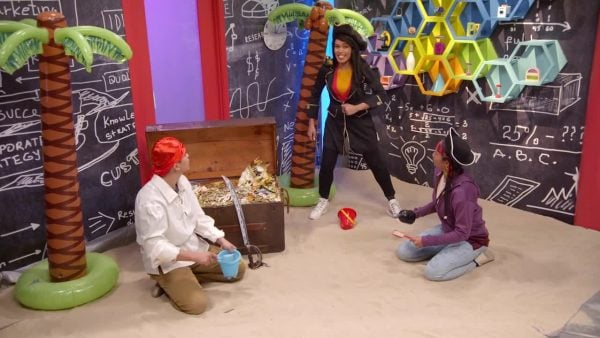Let’s say you find a treasure chest with 497 gold coins. Then, the next day you find a treasure chest with 310 coins. How many coins do you have altogether? We can think of 497 as 4 hundreds, 9 tens, and 7 ones, and 310 as 3 hundreds, 1 ten, and 0 ones. Then we can add by place: 400 + 300 = 700, 90 + 10 = 100, and 7 + 0 = 7, 700 + 100 + 7 = 807. The tens added to 100, making one more hundred, and no tens. The 0 shows there are no tens and that 8 is in the hundreds place. Also, since 497 is so close to 500, we could take 3 from 310 to give to 497, making 500 + 307. This is easier to add since only the hundreds need to be added and can be done mentally.

Counting Treasure Let’s say you find a treasure chest with 497 gold coins. Then, the next day you find a treasure chest with 310 coins. How many coins do you have altogether? We can think of 497 as 4 hundreds, 9 tens, and 7 ones, and 310 as 3 hundreds, 1 ten, and 0 ones. Then we can add by place: 400 + 300 = 700, 90 + 10 = 100, and 7 + 0 = 7, 700 + 100 + 7 = 807. The tens added to 100, making one more hundred, and no tens. The 0 shows there are no tens and that 8 is in the hundreds place. Also, since 497 is so close to 500, we could take 3 from 310 to give to 497, making 500 + 307. This is easier to add since only the hundreds need to be added and can be done mentally.

### Farm Animals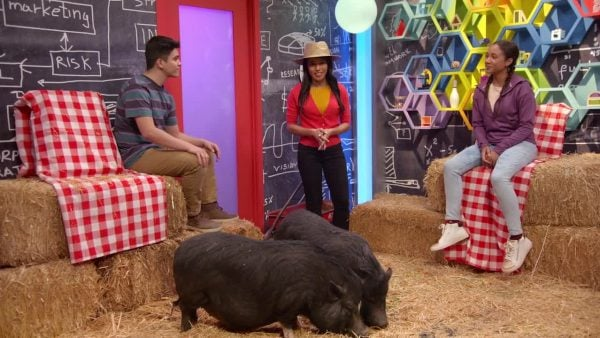Let’s say two farmers raise pigs for the county fair. The first pig weighs 257 kg and the other farmer’s pig weighs 142 kg. How much more does the first pig weigh than the second pig? We can write each number by place and then subtract. 257 is 200 + 50 + 7, and 142 is 100 + 40 + 2. Now we can subtract each place: 200 - 100 = 100, 50 - 40 = 10, and 7 - 2 = 5. 100 + 10 + 5 = 115, so the larger pig weighs 115 kilograms more than the smaller pig. Or, we can use a number line. We can start at the lesser number, 142, then jump forward to 257. First we’ll jump 100 to 242. Then we can jump 10 to 252, and then 5 to 257. Altogether we jumped 100 + 10 + 5, or 115.

Farm Animals Let’s say two farmers raise pigs for the county fair. The first pig weighs 257 kg and the other farmer’s pig weighs 142 kg. How much more does the first pig weigh than the second pig? We can write each number by place and then subtract. 257 is 200 + 50 + 7, and 142 is 100 + 40 + 2. Now we can subtract each place: 200 - 100 = 100, 50 - 40 = 10, and 7 - 2 = 5. 100 + 10 + 5 = 115, so the larger pig weighs 115 kilograms more than the smaller pig. Or, we can use a number line. We can start at the lesser number, 142, then jump forward to 257. First we’ll jump 100 to 242. Then we can jump 10 to 252, and then 5 to 257. Altogether we jumped 100 + 10 + 5, or 115.

### Rock Concert T-shirts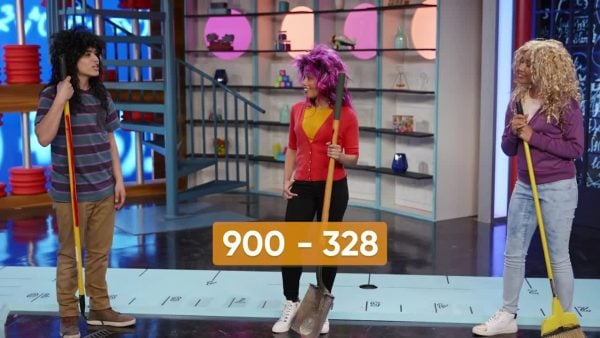Let’s say you have 900 t-shirts to sell at a rock concert. At the end of the concert you have 328 t-shirts left. How many shirts did you sell? To find out how many were sold, you need to find the difference between 900 and 328. Or you can think of the problem as 900 - ___ = 328 or 328 + ___ = 900. Again we can write each number by place value, but this time it’s a little different. There are no tens or ones to subtract, so we can regroup 1 hundred as 10 tens, then one of the tens as 10 ones. So now 900 is 800 + 90 + 10. 328 is 300 + 20 + 8, so now we can subtract. 800 - 300 = 500, 90 - 20 = 70, and 10 - 8 = 2. We have 500 + 70 + 2, or 372. So there are 372 t-shirts left after the concert. We can also use a number line to count up from 328 to 900. Start at 328 and jump 2 to 330. Now jump 70 to 400. Last, jump 500 to 900. We jumped 2 + 70 + 500, or 572. Try this one yourself: Let’s say Greg’s parents buy a computer for \$567, but give the clerk \$600. What will their change be?

Rock Concert T-shirts Let’s say you have 900 t-shirts to sell at a rock concert. At the end of the concert you have 328 t-shirts left. How many shirts did you sell? To find out how many were sold, you need to find the difference between 900 and 328. Or you can think of the problem as 900 - ___ = 328 or 328 + ___ = 900. Again we can write each number by place value, but this time it’s a little different. There are no tens or ones to subtract, so we can regroup 1 hundred as 10 tens, then one of the tens as 10 ones. So now 900 is 800 + 90 + 10. 328 is 300 + 20 + 8, so now we can subtract. 800 - 300 = 500, 90 - 20 = 70, and 10 - 8 = 2. We have 500 + 70 + 2, or 372. So there are 372 t-shirts left after the concert. We can also use a number line to count up from 328 to 900. Start at 328 and jump 2 to 330. Now jump 70 to 400. Last, jump 500 to 900. We jumped 2 + 70 + 500, or 572. Try this one yourself: Let’s say Greg’s parents buy a computer for \$567, but give the clerk \$600. What will their change be?

## ADDITION & SUBTRACTION WITHIN 1,000 VOCABULARY

Friendly Number
An adjusted number that makes an operation easier to do. For example, if we were adding 498 and 342, we could add 2 to 498 to make it 500. The 2 that was added would be taken from 342, making it 340. 500 + 340 is easier to add than 498 + 342.
Combining two or more numbers to find the total.
Subtraction
Finding the difference between two numbers, how far apart they are.
Place Value
The value of each digit in a number. In our number system, each place has a value 10 times more than the place value to the left. The first place is the ones, then the tens, then the hundreds. The places keep going for larger and larger numbers.
Regroup
Converting a place total greater than 10 to 1 of the next place, with the remainder staying in the current place. Or, converting one from a larger place to 10 of the next smaller place. Regrouping is done to keep each place less than 10 when adding, and to make it possible to subtract when the number being subtracted is larger than the other number.
Sum
The result when numbers are added.
The result when numbers are subtracted.

## ADDITION & SUBTRACTION WITHIN 1,000 DISCUSSION QUESTIONS

### How could you add 346 + 432 using base 10 blocks?

I could use base-10 blocks, or a number line. I could start with 3 hundreds, 4 tens and 6 ones to represent 346, and 4 hundreds, 3 tens, and 2 ones to represent 432. Putting the blocks together I would have 7 hundreds, 7 tens, and 8 ones, for a total of 778 blocks.

### How could you add 528 + 297 using friendly numbers?

297 is very close to 300, which would be easier to add to 528 because only the hundreds would change. 297 is 3 away from 300, so I can take 3 from 528, making it 525, and give the 3 to 497, making it 500. Since the 3 is still included, the total won’t change. Now we have 525 + 300. 500 + 300 = 800, with 25 more, or 825.

### How could you subtract 737 – 503 using friendly numbers?

503 is close to 500, which would be easier to subtract from 737. I can take 3 from 503 to make it 500, but I also need to take 3 from 737 so the numbers stay the same amount apart, so 737 becomes 734. Now we have 734 - 500. 700 - 500 = 200, 34 more, or 234.

### How could you subtract 800 – 347?

Since 800 has no tens or ones to subtract we can regroup 1 hundred as 10 tens, and one of the tens as 10 ones. So 800 becomes 700 + 90 + 10. Now we can write 347 as 300 + 40 + 7 and subtract by place value: 700 - 300 = 400, 90 - 40 = 50, and 10 - 7 = 3. So we have 400 + 50 + 3 = 453. We could also count up from 347 to 800 on the number line. First hop 3 from 347 to 350. Then hop 50 to 400, and then hop 400 to 800. Altogether we hopped 3, then 50, then 400, or 453.

### Wendy says that 647 – 438 = 211, is she correct? Explain.

She is not correct. If Wendy was correct then 211 + 438 should be 647. If we add the ones: 1 + 8 = 9, but there is a 7 in the ones place of 647. When Wendy subtracted the ones, she did not see that 8 is greater than 7. She should have regrouped a ten as 10 ones, but looked at the difference between 7 and 8 and got 1.
X

## Success

We’ve sent you an email with instructions how to reset your password.
Ok

## Choose Your Free Trial Period### 7 Days Free TrialContinue to Lessons### 90 Days Free TrialGet 90 days free by inviting other teachers to try it too.

Share with Teachers

## Get 90 Days Free

### By inviting 4 other teachers to try it too.

4 required

Skip, I will use a 7 days free trial

## Thank You!

Enjoy your free 90 days trial